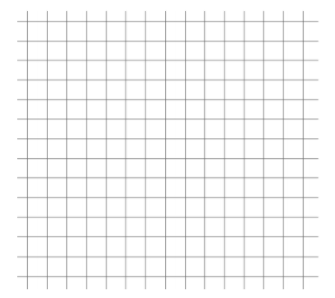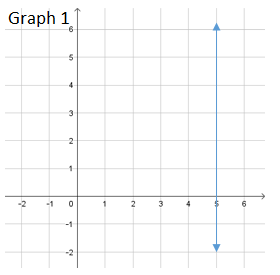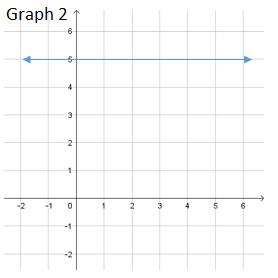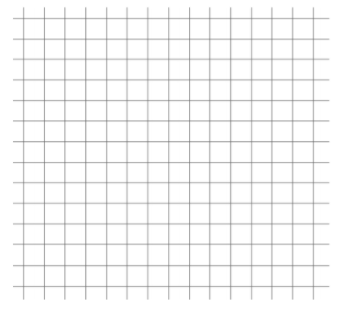# Linear Relationships

## Objective

Write equations into slope-intercept form in order to graph. Graph vertical and horizontal lines.

## Common Core Standards

### Core Standards

?

• 8.EE.B.6 — Use similar triangles to explain why the slope m is the same between any two distinct points on a non-vertical line in the coordinate plane; derive the equation y = mx for a line through the origin and the equation y = mx + b for a line intercepting the vertical axis at b.

?

• 8.EE.C.7

## Criteria for Success

?

1. Distinguish between standard form and slope-intercept form.
2. Rewrite an equation into slope-intercept form by solving for $y$.
3. Graph a linear equation using the slope and $y$-intercept.
4. Understand that the equation $y=a$, where a is a constant, is a horizontal line with a zero slope, and the equation ${x=a}$ is a vertical line with undefined slope.

## Tips for Teachers

?

Lesson 9 is a continuation of Lesson 8 but presents equations in standard form that are then written into slope-intercept form as a strategy to graph.

#### Fishtank Plus

• Problem Set
• Student Handout Editor
• Vocabulary Package

## Anchor Problems

?

### Problem 1

Given the equation ${2x+3y=9}$, determine the slope and $y$-intercept, and then graph the line that represents the equation.### Problem 2

Write the equation below into slope-intercept form and then graph the line that represents the equation.

${-4x-2y=-5}$### Problem 3

Match each equation to a graph. Be prepared to explain your reasoning.

Equation A: ${ y=5}$
Equation B: ${x=5}$## Problem Set

?The following resources include problems and activities aligned to the objective of the lesson that can be used to create your own problem set.

Identify the slope and $y$-intercept of the equation $12x-8y=24$.